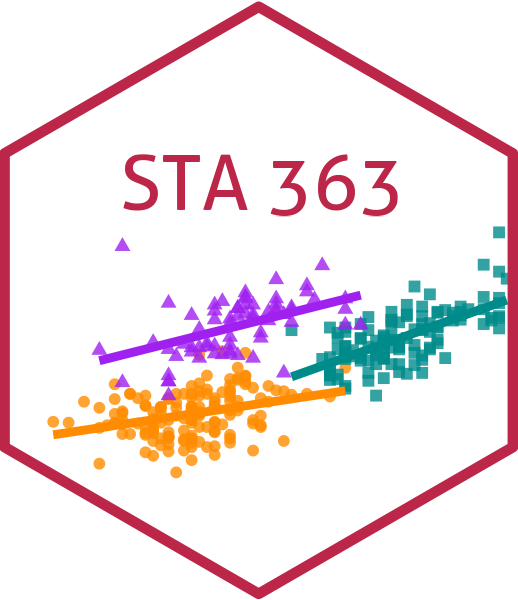# Decision trees - Classification trees

Lucy D’Agostino McGowan

## Classification Trees

• Very similar to regression trees except it is used to predict a qualitative response rather than a quantitative one

• We predict that each observation belongs to the most commonly occuring class of the training observations in a given region

## Fitting classification trees

• We use recursive binary splitting to grow the tree

• Gini index: $G = \sum_{k=1}^K \hat{p}_{mk}(1-\hat{p}_{mk})$

• This is a measure of total variance across the $K$ classes. If all of the $\hat{p}_{mk}$ values are close to zero or one, this will be small

• The Gini index is a measure of node purity small values indicate that node contains predominantly observations from a single class

• In R, this can be estimated using the gain_capture() function.

## Classification tree - Heart Disease Example

• Classifying whether 303 patients have heart disease based on 13 predictors (Age, Sex, Chol, etc)

## 1. Split the data into a cross-validation set

heart_cv <- vfold_cv(heart, v = 5)

How many folds do I have?

## 2. Create a model specification that tunes based on complexity, $\alpha$

tree_spec <- decision_tree(
cost_complexity = tune(),
tree_depth = 10,
mode = "classification") %>%
set_engine("rpart")

wf <- workflow() |>
recipe(HD ~ Age + Sex + ChestPain + RestBP + Chol + Fbs +
RestECG + MaxHR + ExAng + Oldpeak + Slope + Ca,
data = heart
)
) |>
add_model(tree_spec)

## 3. Fit the model on the cross validation set

grid <- expand_grid(cost_complexity = seq(0.01, 0.05, by = 0.01))
model <- tune_grid(wf,
grid = grid,
resamples = heart_cv,
metrics = metric_set(gain_capture, accuracy)) 

What $\alpha$s am I trying?

## 5. Choose $\alpha$ that minimizes the Gini Index

best <- model %>%
select_best(metric = "gain_capture")

## 6. Fit the final model

final_wf <- wf |>
finalize_workflow(best)

final_model <- fit(final_wf, data = heart)

## 7. Examine how the final model does on the full sample

final_model %>%
predict(new_data = heart) %>%
bind_cols(heart) %>%
conf_mat(truth = HD, estimate = .pred_class) %>%
autoplot(type = "heatmap")

## Decision trees

### Pros

• simple
• easy to interpret

### Cons

• not often competitive in terms of predictive accuracy
• Next we will discuss how to combine multiple trees to improve accuracy